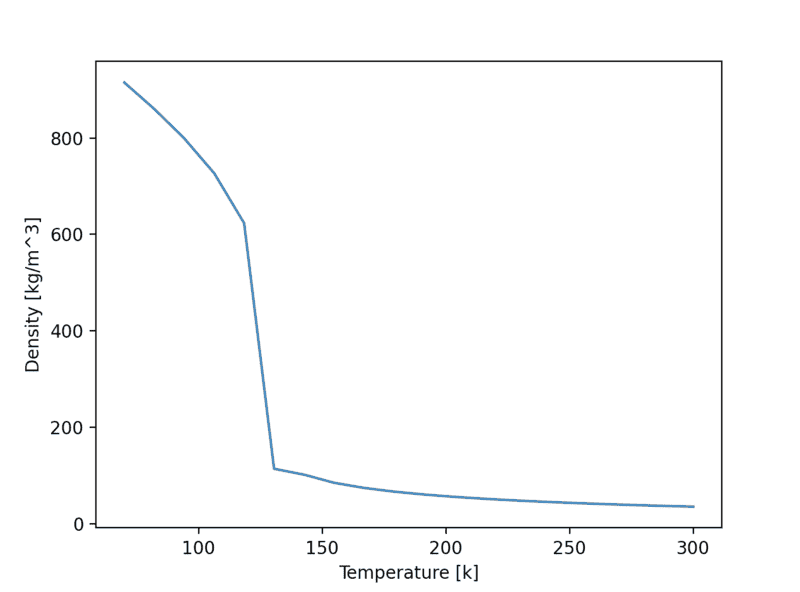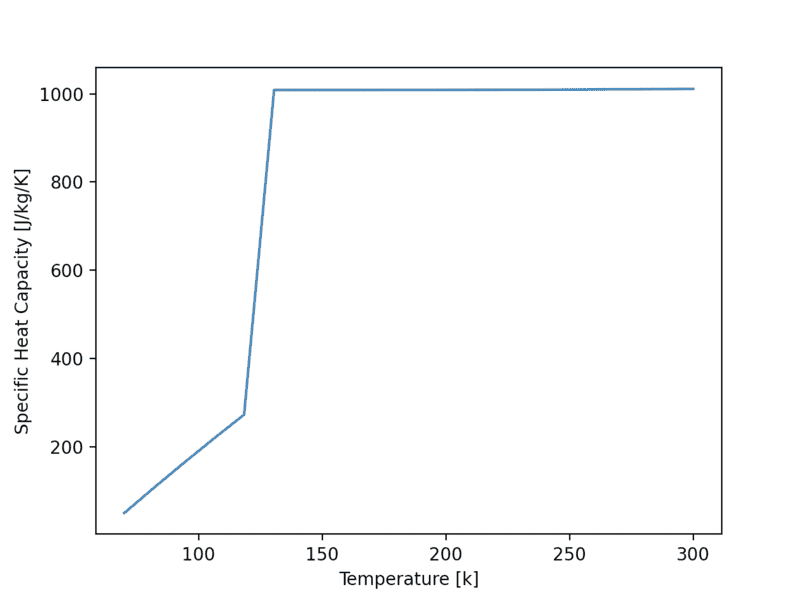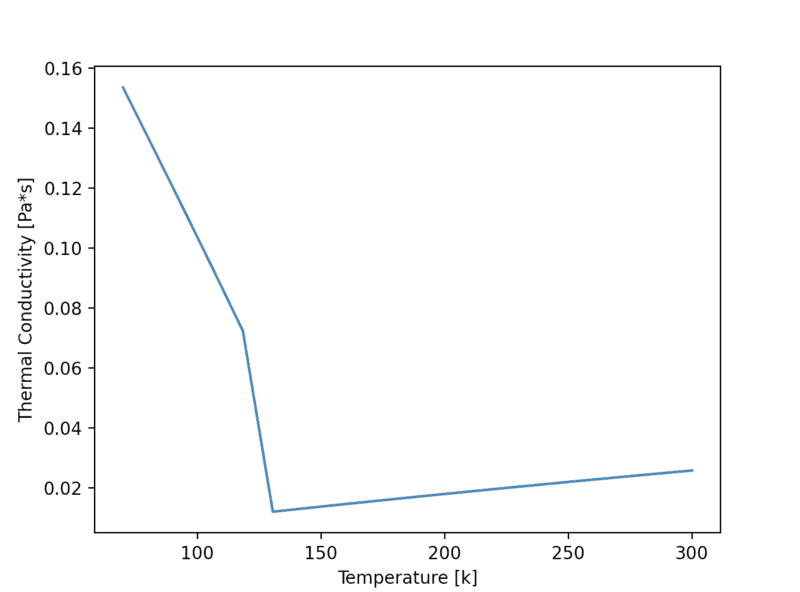Modelling of two phase flow in packed bed using conservation equations

Chestermiller
Mentor
Yes I actually started Denbigh yesterday and it seems to have similar stuff, so I might continue with this (the effect of composition on liquid heat capacity, deviation from Raoults law). Is this reasonable?

The latter, just the ideal gas heat capacity (function of temperature only). I think I can check the variation of liquid heat capacity with temperature for a given pressure, though.

So the plan is loosely to tackle the non ideality of the vapour and liquid phases? And yes I agree that this will require textbook reading on my part, for me to be able to discuss this (two days or so of reading)
The heat capacity is a function of pressure also (for non-ideal gas behavior). You really need to include the effect of pressure on gas enthalpy.

The heat capacity is a function of pressure also (for non-ideal gas behavior). You really need to include the effect of pressure on gas enthalpy.
HI Chet, yes there is h(T,P) functionality in the thermo library so I can use that

In regards to the non-ideality of air, yes as you said the vapour is definitely non-ideal, and actually it seems at lower temperatures air is increasingly non-ideal i.e. liquid phase is even more non-ideal. Increasing the pressure results in an increase in non-ideality also. So yes its safe to say vapour and liquid phase air are both non-ideal

The functionality is absolutely there to model this. There is a bit of setup work required in python to get the actual activity coefficients. I'll do that to get an exact idea of how non-ideal air is at various temperatures.

So in terms of implementing non-ideal air, how can we do this in stages? Are these reasonable as a first two?

1) Implement the Ergun equation in the ideal model, to show the pressure decreasing across the system (wont affect any parameter except mass as this is the only place we use P). I have this implemented in a separate script, just need to edit it to find the pressure drop at any point in the system
2) add non ideal correlations for density and heat capacity (as a function of T and P)

Chestermiller
Mentor
HI Chet, yes there is h(T,P) functionality in the thermo library so I can use that

In regards to the non-ideality of air, yes as you said the vapour is definitely non-ideal, and actually it seems at lower temperatures air is increasingly non-ideal i.e. liquid phase is even more non-ideal. Increasing the pressure results in an increase in non-ideality also. So yes its safe to say vapour and liquid phase air are both non-ideal
I'm not so sure it is unreasonable to treat the liquid phase as ideal. This would certainly simplify things greatly. I suggest checking the literature relating to the VLE behavior of O2-N2 liquid mixtures and the activity coefficients for such mixtures.
The functionality is absolutely there to model this. There is a bit of setup work required in python to get the actual activity coefficients. I'll do that to get an exact idea of how non-ideal air is at various temperatures.

So in terms of implementing non-ideal air, how can we do this in stages? Are these reasonable as a first two?
There is certainly going to be data on the activity coefficients for O2 and N2 in the vapor phase. Also, maybe the Lewis-Randle rule applies to the vapor. Spend some time digging in the literature.
1) Implement the Ergun equation in the ideal model, to show the pressure decreasing across the system (wont affect any parameter except mass as this is the only place we use P). I have this implemented in a separate script, just need to edit it to find the pressure drop at any point in the system
2) add non ideal correlations for density and heat capacity (as a function of T and P
Once you have the non-ideal PVT behavior of the vapor mixtures, you can, knowing the ideal gas heat capacities of N2 and O2 vapor as a function of temperature, get the non-ideal variations in enthalpy with temperature and pressure.

I'm not so sure it is unreasonable to treat the liquid phase as ideal. This would certainly simplify things greatly. I suggest checking the literature relating to the VLE behavior of O2-N2 liquid mixtures and the activity coefficients for such mixtures.
Checking the literature for the O2-N2 liquid mixture VLE behaviour doesn't seem to return much regarding the activity coefficients.

To possibly simplify further I have one question - why not use an existing EOS model rather than the activity coefficients to model the vapour and liquid phases? If we used an EOS (one already written in code) it seems we could calculate any T or P dependent property easily, and we could assume non-ideality for the liquid phase because the work it would add would not cause a significant increase in modelling difficulty

That said, although I very much prefer the idea of using existing functionality rather than hard coding my own, I don't want to move too fast on that and lose understanding myself

What are your thoughts on using a prewritten EOS instead of an activity coefficient model?

Also, maybe the Lewis-Randle rule applies to the vapor.
Once you have the non-ideal PVT behavior of the vapor mixtures, you can, knowing the ideal gas heat capacities of N2 and O2 vapor as a function of temperature, get the non-ideal variations in enthalpy with temperature and pressure.
So yes back the EOS point, the thermo library in Python has existing functionality to calculate both ideal and non-ideal heat capacities of pure O2/N2 and mixtures of these. It can also get the non-ideal enthalpy(T,P)

I think we could save lots of time, and add more complex functionality if we use this. Do you think it is reasonable to use this functionality rather than hard code our own?

EDIT: Also, I'm working on getting the activity coefficients for air plotted as a function of temperature using this thermo library. I will post this plot once its working

Chestermiller
Mentor
Checking the literature for the O2-N2 liquid mixture VLE behaviour doesn't seem to return much regarding the activity coefficients.

To possibly simplify further I have one question - why not use an existing EOS model rather than the activity coefficients to model the vapour and liquid phases? If we used an EOS (one already written in code) it seems we could calculate any T or P dependent property easily, and we could assume non-ideality for the liquid phase because the work it would add would not cause a significant increase in modelling difficulty

That said, although I very much prefer the idea of using existing functionality rather than hard coding my own, I don't want to move too fast on that and lose understanding myself

What are your thoughts on using a prewritten EOS instead of an activity coefficient model?

So yes back the EOS point, the thermo library in Python has existing functionality to calculate both ideal and non-ideal heat capacities of pure O2/N2 and mixtures of these. It can also get the non-ideal enthalpy(T,P)

I think we could save lots of time, and add more complex functionality if we use this. Do you think it is reasonable to use this functionality rather than hard code our own?

EDIT: Also, I'm working on getting the activity coefficients for air plotted as a function of temperature using this thermo library. I will post this plot once its working
I looked at the reference, but I don't exactly see how they do this. I assume they have an appropriate data base for pure o2 and n2, and somehow mixture parameters. I guess I'll leave it up to you to figure how to work with this software to get the needed thermodynamic functionalities.

I looked at the reference, but I don't exactly see how they do this. I assume they have an appropriate data base for pure o2 and n2, and somehow mixture parameters. I guess I'll leave it up to you to figure how to work with this software to get the needed thermodynamic functionalities.
Exactly yes there is appropriate data taken from a number of sources (DIPPR, Perrys, etc) to allow for pure and mixture parameter calculations.

I can work with the software absolutely (I have used it for the dew/bubble and Ergun calculations).

I could start with say some plots of air heat capacity and density for our range of temperatures, for a few pressures? To get an idea of how we would expect the model output to change by adding these two in. I'll add them in separately then

Chestermiller
Mentor
Exactly yes there is appropriate data taken from a number of sources (DIPPR, Perrys, etc) to allow for pure and mixture parameter calculations.

I can work with the software absolutely (I have used it for the dew/bubble and Ergun calculations).

I could start with say some plots of air heat capacity and density for our range of temperatures, for a few pressures? To get an idea of how we would expect the model output to change by adding these two in. I'll add them in separately then
What we really need is temperature and density (or specific volume) as functions of enthalpy and pressure for an overall mixture of 79% n2 and 21% o2 (including the two phase region). Starting from enthalpy and density as functions of temperature and pressure for such an overall mixture.

What we really need is temperature and density (or specific volume) as functions of enthalpy and pressure for an overall mixture of 79% n2 and 21% o2 (including the two phase region). Starting from enthalpy and density as functions of temperature and pressure for such an overall mixture.
To confirm, we are looking to get to this?
$$T(H,P)$$ $$\rho(T,P)$$

And we're assuming we will have this available to start:
$$H(T,P)$$ $$\rho(T,P)$$

So we would need to 'invert' these functions to get to the objective functions?

I can check to see if the goal functions already exist just in case

Last edited:
•Chestermiller
What we really need is temperature and density (or specific volume) as functions of enthalpy and pressure for an overall mixture of 79% n2 and 21% o2 (including the two phase region). Starting from enthalpy and density as functions of temperature and pressure for such an overall mixture.
Just as a side note - here are the plots mentioned earlier, 79%/21% air for a pressure of 30 bar:

Density:Heat capacity:Thermal conductivity (included this in case we eventually split U into components):Last edited:
What we really need is temperature and density (or specific volume) as functions of enthalpy and pressure for an overall mixture of 79% n2 and 21% o2 (including the two phase region). Starting from enthalpy and density as functions of temperature and pressure for such an overall mixture.
Ahh ok it just clicked with me now why we need those specifically. To replace the temperature and mass functions we've got currently implemented. Got it

•Chestermiller
Ahh ok it just clicked with me now why we need those specifically. To replace the temperature and mass functions we've got currently implemented. Got it
Hi Chet, away for 1 week on annual leave. Will update here once I'm back

•Chestermiller